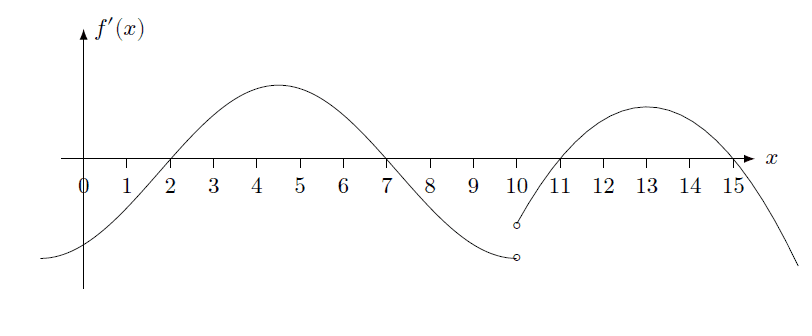# Determine the relative maximum and minimum on the graph

• MHB
jaychay
Given that f is the function on (−∞, ∞) and the graph is the derivative of f

1.) Find the critical point on the graph ?
2.) Find the interval of the increasing function on the graph ?
3.) Find the interval of the decreasing function on the graph ?
4.) Find the point which is the absolute maximum on the graph ?
5.) Find the point which is the absolute minimum on the graph ?jaychay
Given that f is the function on (−∞, ∞) and the graph is the derivative of f

1.) Find the critical point on the graph ?
2.) Find the interval of the increasing function on the graph ?
3.) Find the interval of the decreasing function on the graph ?
4.) Find the point which is the absolute maximum on the graph ?
5.) Find the point which is the absolute minimum on the graph ?

View attachment 10704
Do the graph have the relative maximum and relative minimum ?

Homework Helper
MHB
Let's add the graph of $\color{red}f(x)$ at an arbitrary level. That is, let's pick $\color{red}f(0)=0$.
And let's assume that $\color{red}f(x)$ is continuous at $x=10$.

\begin{tikzpicture}[
declare function={
df1(\x) = 2*cos(3/11*360)-2*cos((\x+1)/11*360);
df2(\x) = 1.25-5/16*(\x-13)^2;
f1(\x) = 2*cos(3/11*360)*\x-2*(sin((\x+1)/11*360) - sin(1/11*360))*11/(2*pi);
f2(\x) = 1.25*(\x-10)-5/16*((\x-13)^3 + 27)/3+f1(10);
}]
%\draw[help lines] (-1,-3) grid (16,4);
\draw[-latex] (-1,0) -- (16,0);
\draw[-latex] (0,-3) -- (0,4);
\draw foreach \i in {1,...,15} { (\i,0.1) -- (\i,-0.1) node[below] {$\i$} };
% \draw foreach \i in {-2,...,2} { (0.1,\i) -- (-0.1,\i) node[ left ] {$\i$} };
\draw[domain=-1:10, variable=\x, thick, smooth] plot ({\x}, {df1(\x)}) (3, {df1(3)}) node[above left] {$f'(x)$};
\draw[domain=10:16, variable=\x, thick, smooth] plot ({\x}, {df2(\x)});
\filldraw[fill=black!5, thick] (10,{df1(10)}) circle (0.05) (10,{df2(10)}) circle (0.05);
\draw[domain=-1:10, variable=\x, red, thick, smooth] plot ({\x}, {f1(\x)}) (9, {f1(9)}) node[above right] {$f(x)$};;
\draw[domain=10:16, variable=\x, red, thick, smooth] plot ({\x}, {f2(\x)});
\end{tikzpicture}

Can we find those points and intervals now?

jaychay
Let's add the graph of $\color{red}f(x)$ at an arbitrary level. That is, let's pick $\color{red}f(0)=0$.
And let's assume that $\color{red}f(x)$ is continuous at $x=10$.

\begin{tikzpicture}[
declare function={
df1(\x) = 2*cos(3/11*360)-2*cos((\x+1)/11*360);
df2(\x) = 1.25-5/16*(\x-13)^2;
f1(\x) = 2*cos(3/11*360)*\x-2*(sin((\x+1)/11*360) - sin(1/11*360))*11/(2*pi);
f2(\x) = 1.25*(\x-10)-5/16*((\x-13)^3 + 27)/3+f1(10);
}]
%\draw[help lines] (-1,-3) grid (16,4);
\draw[-latex] (-1,0) -- (16,0);
\draw[-latex] (0,-3) -- (0,4);
\draw foreach \i in {1,...,15} { (\i,0.1) -- (\i,-0.1) node[below] {$\i$} };
% \draw foreach \i in {-2,...,2} { (0.1,\i) -- (-0.1,\i) node[ left ] {$\i$} };
\draw[domain=-1:10, variable=\x, thick, smooth] plot ({\x}, {df1(\x)}) (3, {df1(3)}) node[above left] {$f'(x)$};
\draw[domain=10:16, variable=\x, thick, smooth] plot ({\x}, {df2(\x)});
\filldraw[fill=black!5, thick] (10,{df1(10)}) circle (0.05) (10,{df2(10)}) circle (0.05);
\draw[domain=-1:10, variable=\x, red, thick, smooth] plot ({\x}, {f1(\x)}) (9, {f1(9)}) node[above right] {$f(x)$};;
\draw[domain=10:16, variable=\x, red, thick, smooth] plot ({\x}, {f2(\x)});
\end{tikzpicture}

Can we find those points and intervals now?

My answers for all of the question that I have done on my own is
• 1.) 4.5,13
• 2.) [0,4.5] , (10,13]
• 3.) [4.5,10), [13, - ∞)
• 4.) 4.5,13
• 5.) 10
I want to check that I am doing it correct or not because I am not good at discontinuous graph

Homework Helper
MHB
Given that f is the function on (−∞, ∞) and the graph is the derivative of f

1.) Find the critical point on the graph ?
• 1.) 4.5,13

A critical point is a point in the domain of the function where the function is either not differentiable or the derivative is equal to zero.

That's not the case for x=4.5 or x=13 is it?
For which x-values is $f'(x)$ either zero or undefined?

2.) Find the interval of the increasing function on the graph ?
3.) Find the interval of the decreasing function on the graph ?
• 2.) [0,4.5] , (10,13]
• 3.) [4.5,10), [13, - ∞)

A function is increasing if its derivative is greater than zero.
Where is $f'(x)$ greater than zero?

We can also look at the graph for $f(x)$ that I added.
Where is that graph increasing?

4.) Find the point which is the absolute maximum on the graph ?
5.) Find the point which is the absolute minimum on the graph ?
• 4.) 4.5,13
• 5.) 10

We have relative maxima and minima if $f'(x)=0$.
For which x-values is $f'(x)$ equal to zero?
Can we tell whether they correspond to maxima or minima?

jaychay
A critical point is a point in the domain of the function where the function is either not differentiable or the derivative is equal to zero.

That's not the case for x=4.5 or x=13 is it?
For which x-values is $f'(x)$ either zero or undefined?

A function is increasing if its derivative is greater than zero.
Where is $f'(x)$ greater than zero?

We can also look at the graph for $f(x)$ that I added.
Where is that graph increasing?

We have relative maxima and minima if $f'(x)=0$.
For which x-values is $f'(x)$ equal to zero?
Can we tell whether they correspond to maxima or minima?
Thank you very much